# Bayesian Survival Models¶

In order to implement survival models (or any other type of model) in a Bayesian framework, it is important to be aware of the Three Easy Steps in Bayesian modeling:

1. Specify a full probability model
2. Calculate the posterior distribution of the model
3. Check the model

If any of these steps are omitted, you are not doing Bayesian modeling!

## Binomial Model¶

Rat tumor data from Tarone (1982). Data come from 71 experiments conducted with rats. $n_j$ is the total number of rats in the $j$th experiment and $y_j$ is the number of rats that develop a tumor during the experiment.

In :
y = 0,0,0,0,0,0,0,0,0,0,0,0,0,0,1,1,1,1,1,1,1,1,3,2,2,2,2,2,2,2,2,2,1,5,2, \
5,2,7,7,3,3,2,9,10,4,4,4,4,4,4,4,10,4,4,4,5,11,12,5,5,6,5,6,6,6,6,16,15,15,9,4

N = 20,20,20,20,20,20,20,19,19,19,19,18,18,17,20,20,20,20,19,19,18,18,27,25,24,23,20,20,20,20,20,20,10,49,19,46,17, \
49,47,20,20,13,48,50,20,20,20,20,20,20,20,48,19,19,19,22,46,49,20,20,23,19,22,20,20,20,52,46,47,24,14


## Two Extremes: Pooled and Unpooled¶

The simplst model is one in which we pool all the experiments, which implies that all the experiments are measuring the exact same quantity: a single, global probability of tumor development.

The sampling distribution (likelihood) in this case is binomial:

$$y_i \sim \text{Bin}(N_i, p)$$

The unknown quantity is the probability $p$, to which we can ascribe a flat Beta prior:

$$p \sim \text{Beta}(1, 1)$$

Note that a beta distribution with both scale and shape parameters equal to one is the equivalent of a uniform distribution on the unit interval.

In this example, calculating the posterior distribution is easy. The beta distribution is a conjugate prior for the binomial proportion. This means that the posterior distribution is also a beta distribution.

$$Pr(p | y, n) \propto L(y | p, n) Pr(p) \rightarrow \text{Beta} = \text{Binomial}\times\text{Beta}$$

Specifically, for a beta prior distribution with parameters $\alpha$ and $\beta$, the posterior distribution is:

$$\text{Beta}(y+\alpha-1, n-y+\beta-1)$$

Hence, the posterior estimates of alpha an beta for this dataset are:

In :
alpha = sum(y) + 1 - 1
beta = sum(N) - sum(y) + 1 - 1

alpha, beta

Out:
(267, 1472)
In :
1.*alpha/(alpha+beta)

Out:
0.1535365152386429
In :
from pymc import beta_like

x = linspace(0, 1, 1000)
plot(x, [exp(beta_like(i, alpha, beta)) for i in x])
plot(array(y, dtype=float)/array(N), ones(len(y))+abs(random.randn(len(y)))*0.7, 'ro')
xlim(0, 0.5)

Out:
(0, 0.5)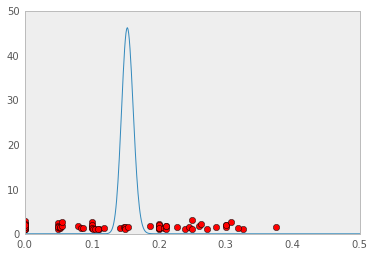The directed acyclic graph (DAG) of this simple model:

In :
from IPython.display import Image
Image(url="http://f.cl.ly/items/00093p0d243n0H1r3T0W/pooled.png")

Out:In :
from pymc import Binomial, Beta, Normal, MCMC, Matplot, Lambda, observed, binomial_like


Here is the prior on $p$:

In :
p = Beta('p', 1, 1, value=0.5)

In :
p.parents

Out:
{'alpha': 1, 'beta': 1}
In :
p.value

Out:
array(0.5)
In :
p.logp

Out:
-4.440892098500626e-16

Now the sampling distribution:

In :
ps = [p]*len(y)
tumor = Binomial('tumor', n=N, p=ps, value=y, observed=True)


In order to do model checking, we will also simulate some data from our model:

In :
tumor_pred = Binomial('tumor_pred', n=N, p=ps, value=y)


We now can create an MCMC object, and sample from it:

In :
M_pooled = MCMC([p, tumor, tumor_pred])
M_pooled.sample(15000, burn=10000)

[****************100%******************]  15000 of 15000 complete

In :
Matplot.plot(p)

Plotting p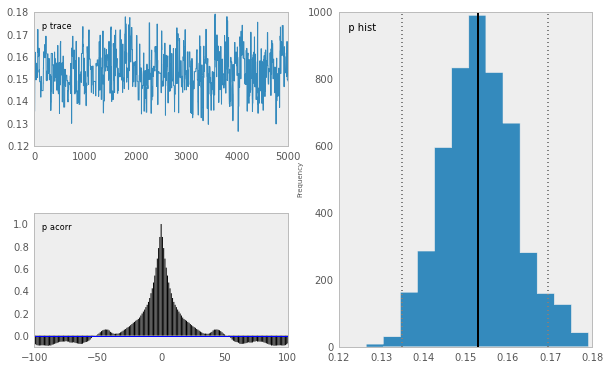### Checking model fit¶

In :
Matplot.gof_plot(tumor_pred.trace(), y, bins=10)

Plotting MCMC-gof
Plotting MCMC-gof
Plotting MCMC-gof
Plotting MCMC-gof
Plotting MCMC-gof
Plotting MCMC-gof
Plotting MCMC-gof
Plotting MCMC-gof
Plotting MCMC-gof
Plotting MCMC-gof
Plotting MCMC-gof
Plotting MCMC-gof
Plotting MCMC-gof
Plotting MCMC-gof
Plotting MCMC-gof
Plotting MCMC-gof
Plotting MCMC-gof
Plotting MCMC-gof
Plotting MCMC-gof
Plotting MCMC-gof
Plotting MCMC-gof
Plotting MCMC-gof
Plotting MCMC-gof
Plotting MCMC-gof
Plotting MCMC-gof
Plotting MCMC-gof
Plotting MCMC-gof
Plotting MCMC-gof
Plotting MCMC-gof
Plotting MCMC-gof
Plotting MCMC-gof
Plotting MCMC-gof
Plotting MCMC-gof
Plotting MCMC-gof
Plotting MCMC-gof
Plotting MCMC-gof
Plotting MCMC-gof
Plotting MCMC-gof
Plotting MCMC-gof
Plotting MCMC-gof
Plotting MCMC-gof
Plotting MCMC-gof
Plotting MCMC-gof
Plotting MCMC-gof
Plotting MCMC-gof
Plotting MCMC-gof
Plotting MCMC-gof
Plotting MCMC-gof
Plotting MCMC-gof
Plotting MCMC-gof
Plotting MCMC-gof
Plotting MCMC-gof
Plotting MCMC-gof
Plotting MCMC-gof
Plotting MCMC-gof
Plotting MCMC-gof
Plotting MCMC-gof
Plotting MCMC-gof
Plotting MCMC-gof
Plotting MCMC-gof
Plotting MCMC-gof
Plotting MCMC-gof
Plotting MCMC-gof
Plotting MCMC-gof
Plotting MCMC-gof
Plotting MCMC-gof
Plotting MCMC-gof
Plotting MCMC-gof
Plotting MCMC-gof
Plotting MCMC-gof
Plotting MCMC-gof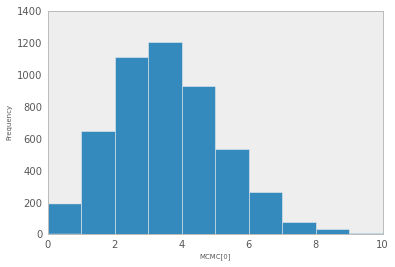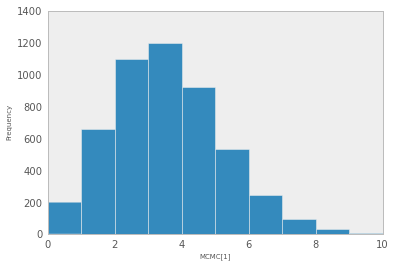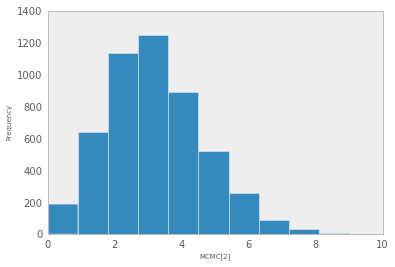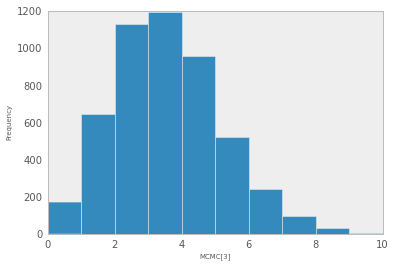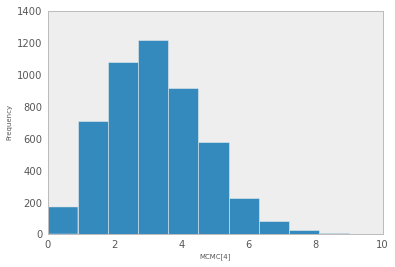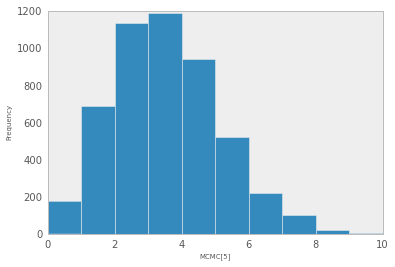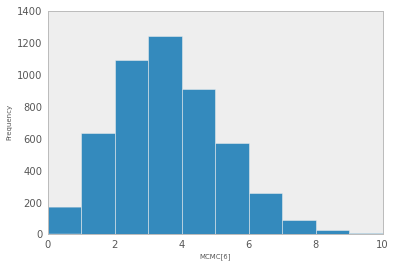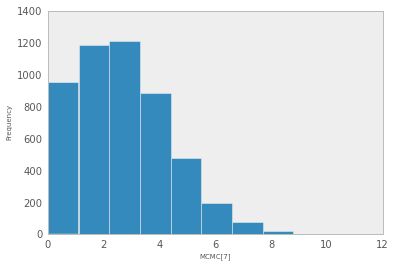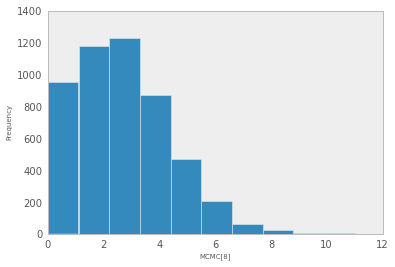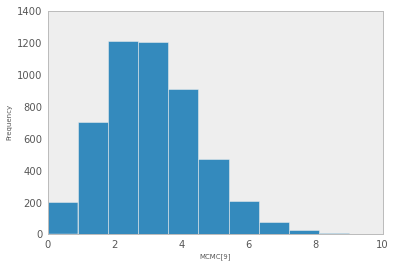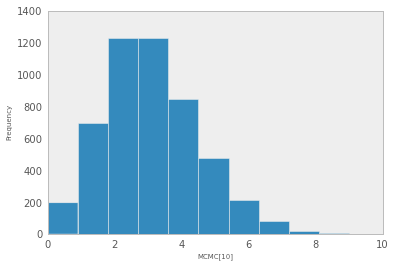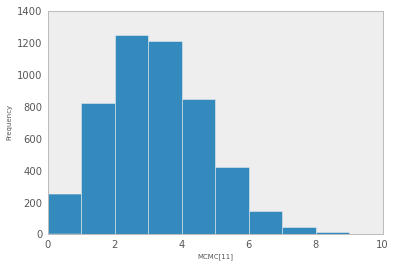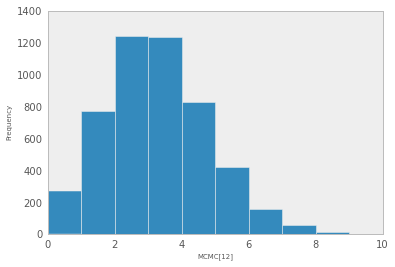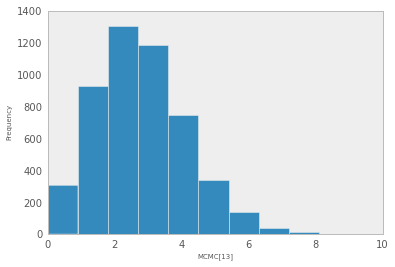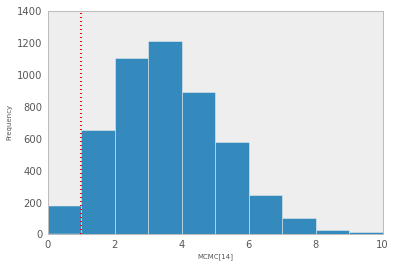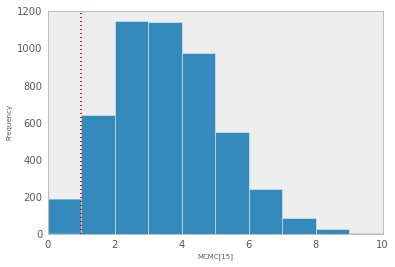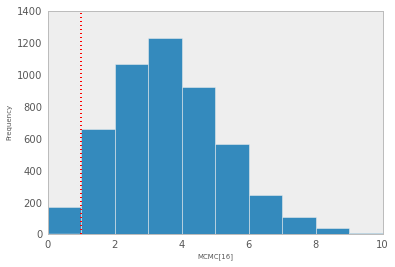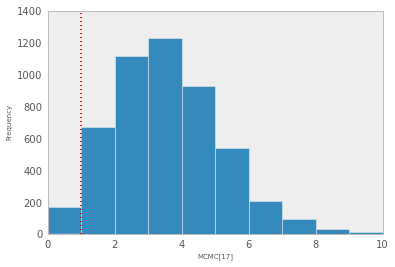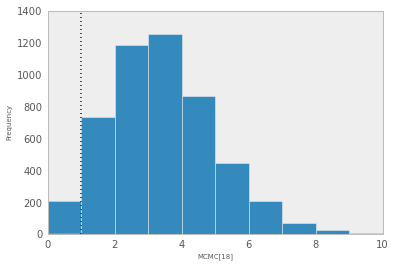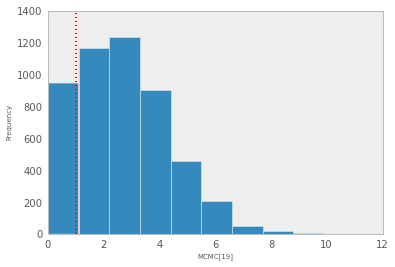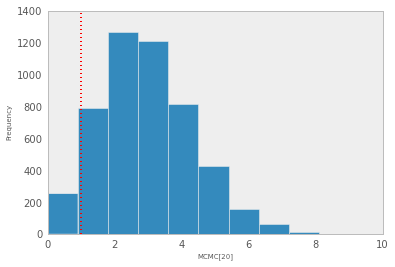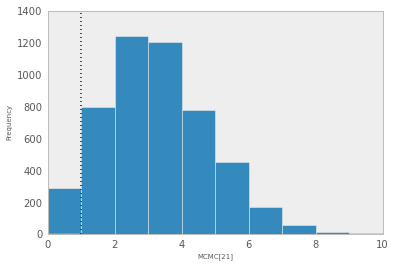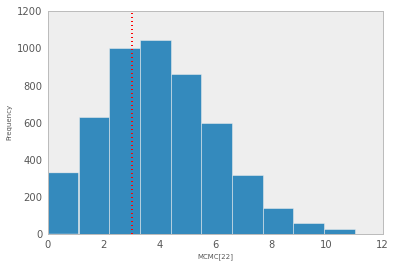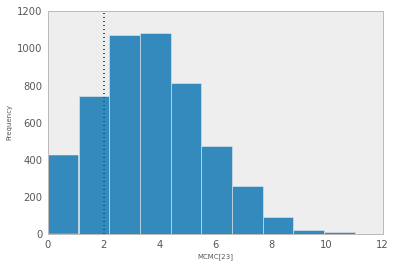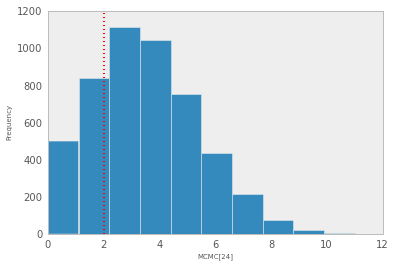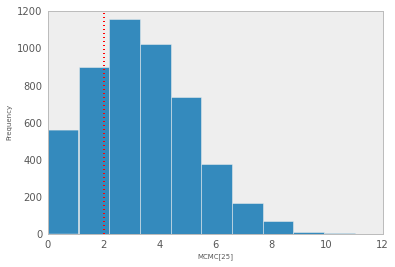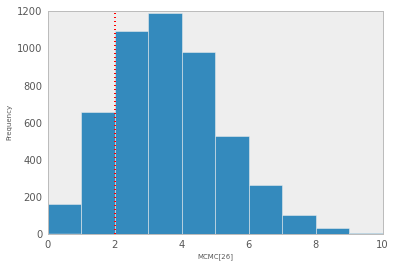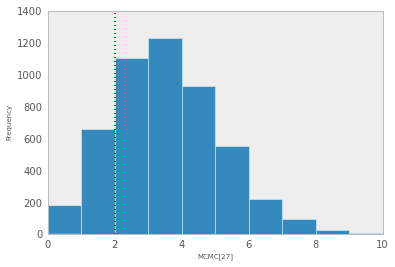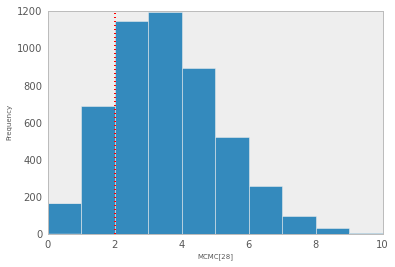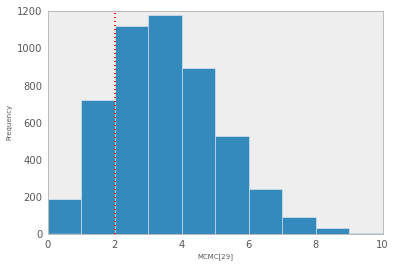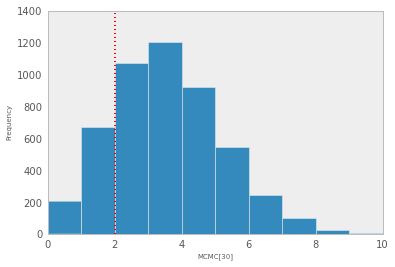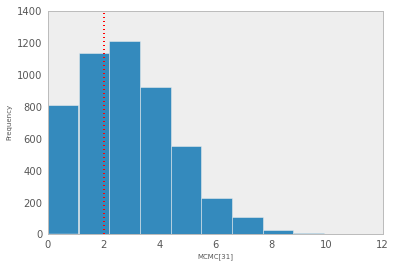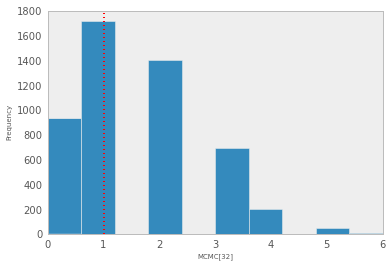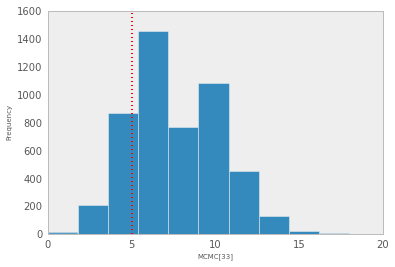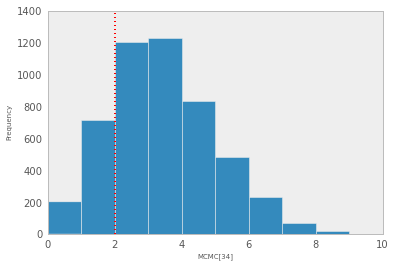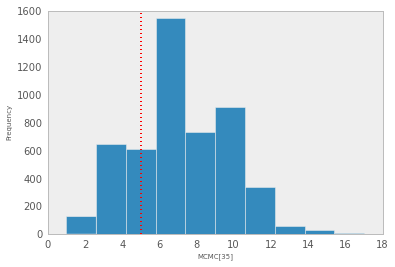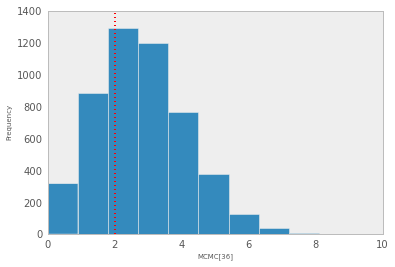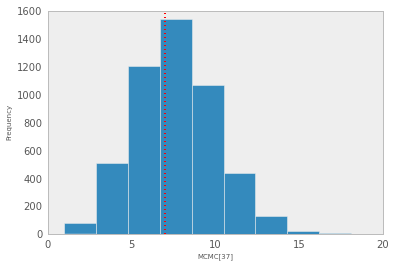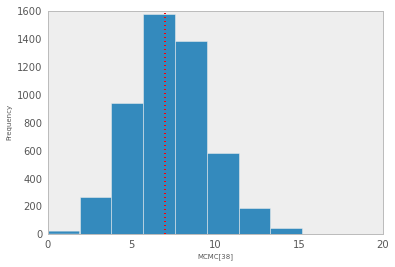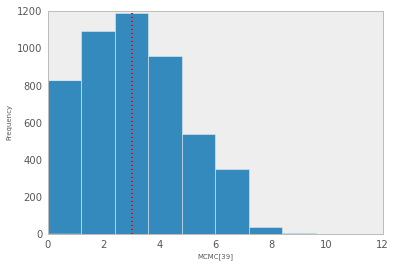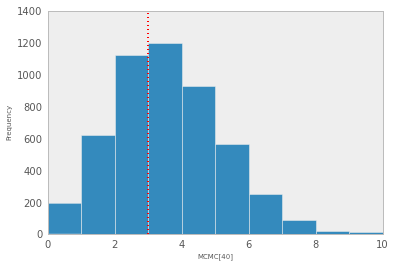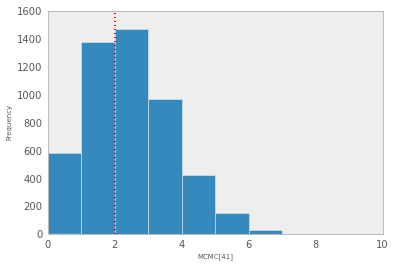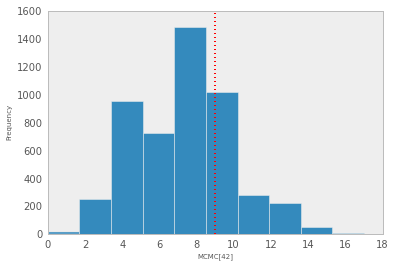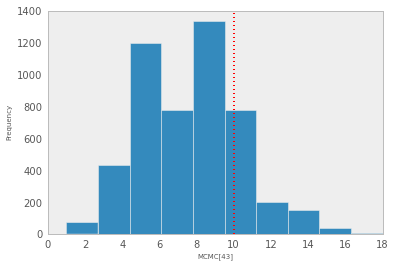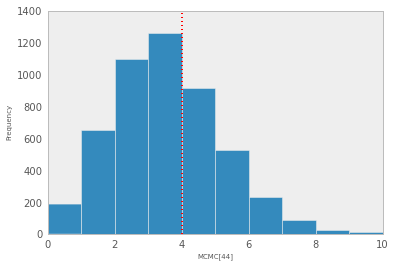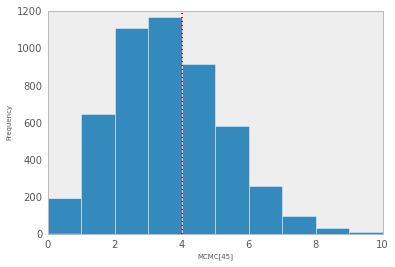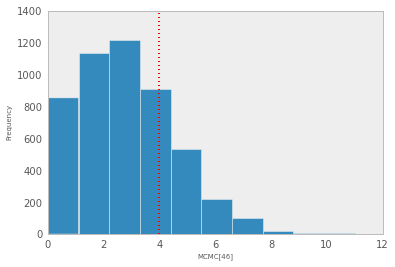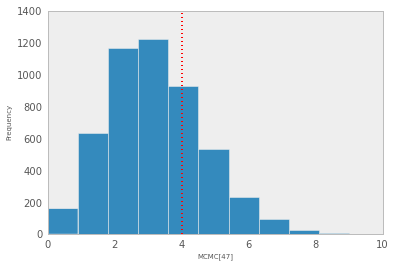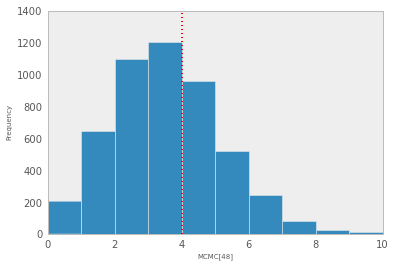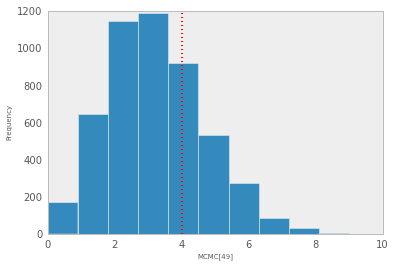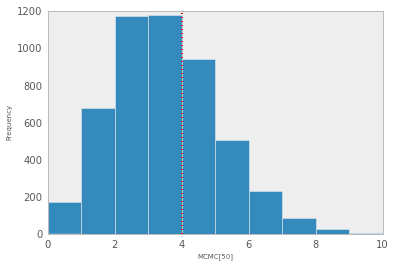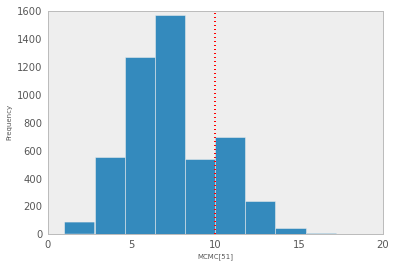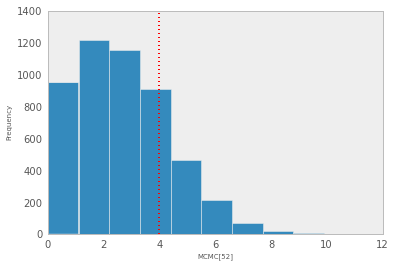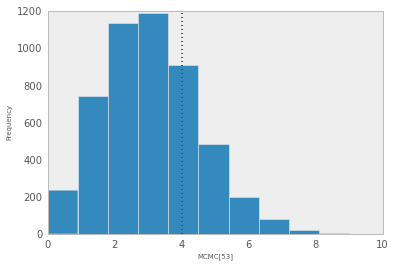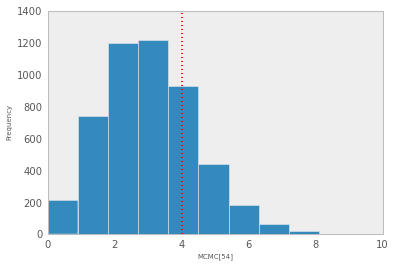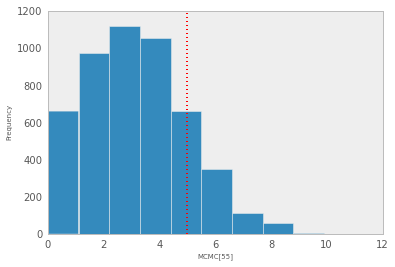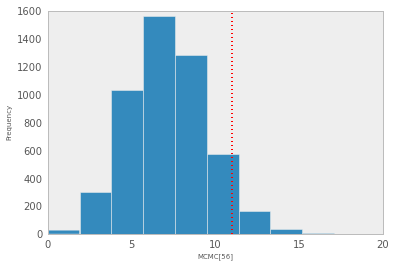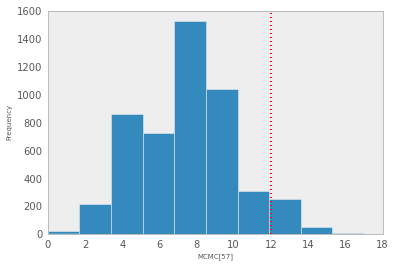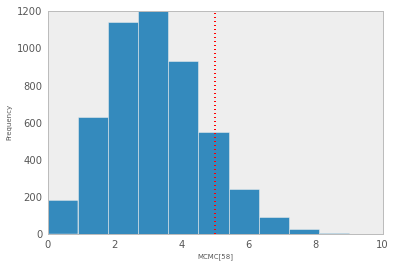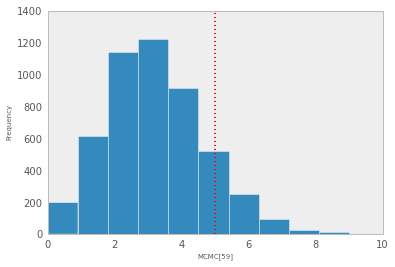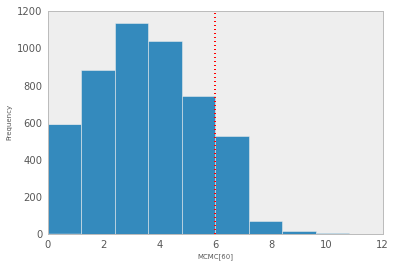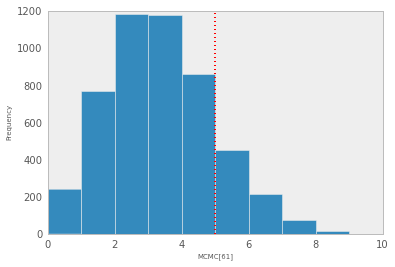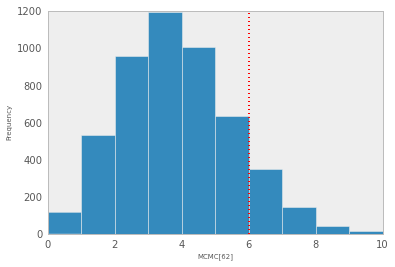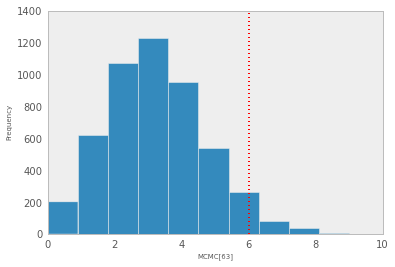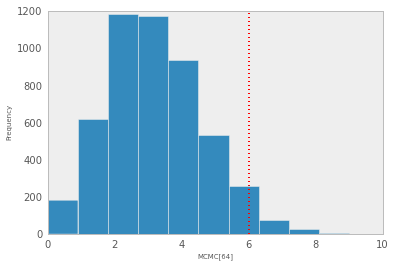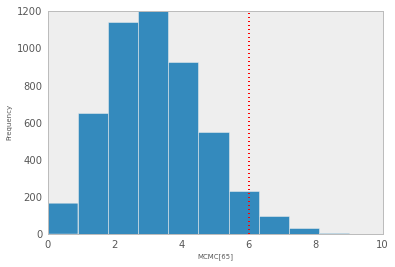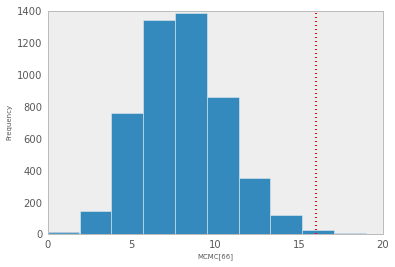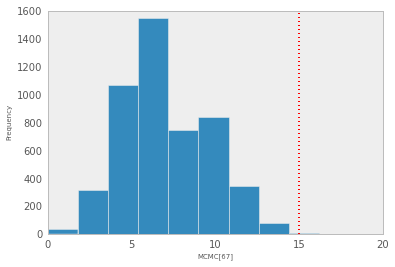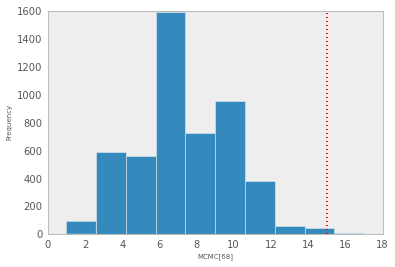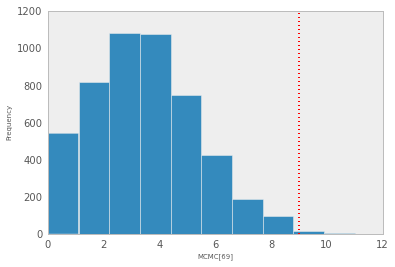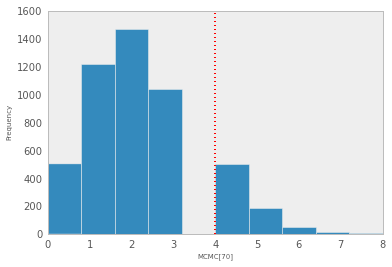### Unpooled Model¶

In :
def unpooled_model(y=y, N=N):

# Prior on probability of tumor
p = [Beta('p_{0}'.format(i), 1, 1) for i in range(len(y))]

# Binomial likelihood
tumor = Binomial('tumor', n=N, p=p, value=y, observed=True)

return locals()

In :
M_unpooled = MCMC(unpooled_model())
M_unpooled.sample(15000, 10000)

[****************100%******************]  15000 of 15000 complete

In :
figure(figsize=(5,8))

Matplot.summary_plot(M_unpooled.p, x_range=(0,0.5))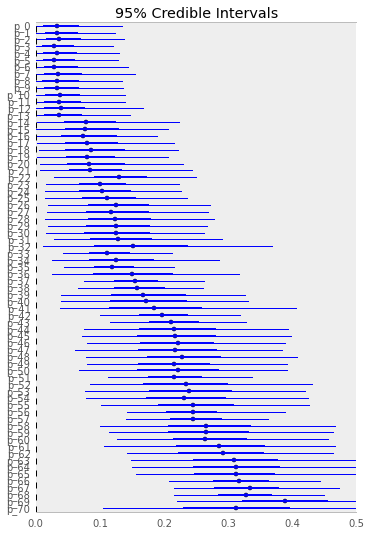## Binomial with Random Effects¶

Obviously, this is a poor model. We have little reason to expect that 71 experiments conducted at different times and places are measuring precisely the same quantity. The "true" value in each case is likely to vary somewhat from the others, due perhaps to variables that we have not measured. In this case, we can think of estimating distinct, but related, parameters; perhaps parameters that can be thought of as coming from a "population" of similar experiments. The effect of a random effect model is to partially pool the data from replicates of similar experiments.

How do we implement this?

Rather than just placing a prior over a global probability $p$, we would like to model a sample of $p$ as coming from some population, as discussed. The easiest way to do this in the context of the model we have already implemented is to use a beta distribution to model the distribution of the probabilities. This distribution has unknown parameters ($\alpha, \beta$) that we need to estimate. So, we need to specify our uncertainty about these parameters using hyper prior distributions. Since the parameters of a beta distribution are positive continuous, a gamma distribution is a convenient choice, but others (e.g. uniform, lognormal) could be used as well.

In :
Image(url="http://f.cl.ly/items/0g1P0p2v2a1t3k321h3A/Screen%20Shot%202013-03-15%20at%209.26.48%20AM.png")

Out:In :
Image(url="http://f.cl.ly/items/0j323X29440X183b0h1K/random_effect.png")

Out:In :
from pymc import rgamma
hist(rgamma(1, 0.01, size=10000), bins=20)

Out:
(array([3335, 2219, 1500,  971,  651,  454,  303,  178,  134,   87,   60,
33,   24,   17,   11,    7,    9,    2,    3,    2]),
array([   0.0027,   41.1899,   82.377 ,  123.5642,  164.7513,  205.9384,
247.1256,  288.3127,  329.4998,  370.687 ,  411.8741,  453.0612,
494.2484,  535.4355,  576.6227,  617.8098,  658.9969,  700.1841,
741.3712,  782.5583,  823.7455]),
<a list of 20 Patch objects>)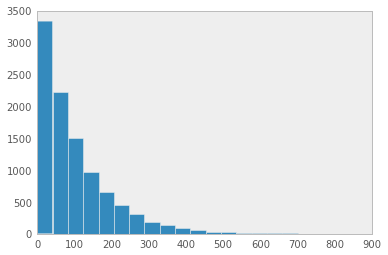In :
from pymc import Gamma

def random_effect_model(y=y, N=N):

# Hyperpriors
alpha = Gamma('alpha', 1, 0.01, value=1)
beta = Gamma('beta', 1, 0.01, value=1)

# Population distribution of tumor probabilities
p = Beta('p', alpha, beta, value=[0.5]*len(y))

# Binomial likelihood
tumor = Binomial('tumor', n=N, p=p, value=y, observed=True)

return locals()

In :
M_random = MCMC(random_effect_model())
M_random.sample(15000, 10000)

[****************100%******************]  15000 of 15000 complete

In :
figure(figsize=(5,8))
a = M_random.alpha.stats()['mean']
b = M_random.beta.stats()['mean']

Matplot.summary_plot([M_random.p], vline_pos=a/(a+b), x_range=(0,0.5))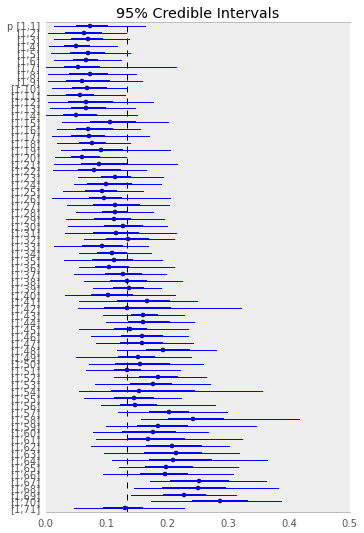We can see that, relative to the unpooled model, estimates are shrunk towards the overall mean.

Clearly, this is a more appropriate fit for the data:

In :
figure(figsize=(5,8))
scatter(array(y, dtype=float)/array(N), range(len(y))[::-1], N)
xlim(0, 0.5)
ylim(0, 71)

Out:
(0, 71)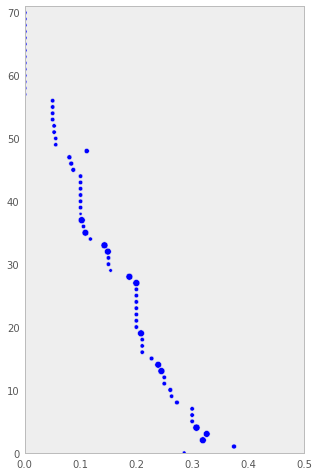# Logistic Model¶

Though the beta-binomial model is mathematically convenient, it can be more difficult to directly interpret the parameters of the population model, a beta distribution. An alternative is to model the mean and variance of the population using, say, a normal model, then use the logit transormation to link it to the binomial sampling model.

In :
from pymc import Lambda, Uniform, invlogit

def logit_model(y=y, N=N):

# Hyperpriors
mu = Normal('mu', 0, 0.001, value=0)
sigma = Uniform('sigma', 0, 1000, value=10)
tau = Lambda('tau', lambda s=sigma: s**-2)

# Population distribution of tumor probabilities
theta = Normal('theta', mu, tau, value=[0.1]*len(y))

# Logit transformation
p = Lambda('p', lambda t=theta: invlogit(t))

# Binomial likelihood
tumor = Binomial('tumor', n=N, p=p, value=y, observed=True)

return locals()

In :
M_logit = MCMC(logit_model())
M_logit.sample(15000, 10000)

[****************100%******************]  15000 of 15000 complete

In :
from pymc import invlogit
hist(invlogit(M_logit.mu.trace()))

Out:
(array([  33,  206,  604, 1109, 1171,  977,  543,  263,   47,   47]),
array([ 0.0919,  0.0994,  0.1069,  0.1144,  0.1219,  0.1294,  0.1369,
0.1444,  0.1519,  0.1594,  0.1669]),
<a list of 10 Patch objects>)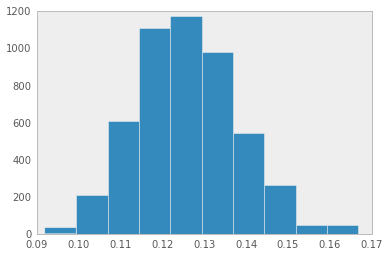In :
figure(figsize=(5,8))

Matplot.summary_plot([M_logit.p], vline_pos=invlogit(M_logit.mu.stats()['mean']), x_range=(0, 0.5))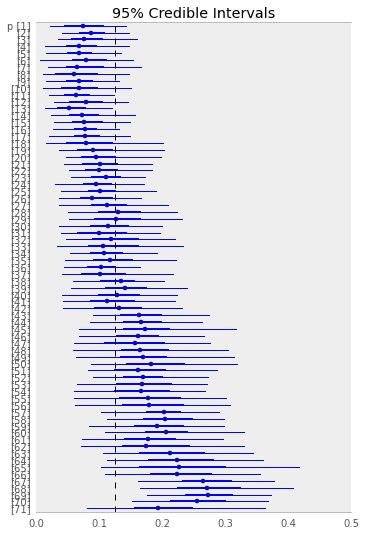## Binary Logistic Model¶

From the Biostats datasets website:

The titanic and titanic2 data frames describe the survival status of individual passengers on the Titanic. The titanic data frame does not contain information from the crew, but it does contain actual ages of half of the passengers. The principal source for data about Titanic passengers is the Encyclopedia Titanica. The datasets used here were begun by a variety of researchers. One of the original sources is Eaton & Haas (1994) Titanic: Triumph and Tragedy, Patrick Stephens Ltd, which includes a passenger list created by many researchers and edited by Michael A. Findlay.

The variables on our extracted dataset are pclass, survived, name, age, embarked, home.dest, room, ticket, boat, and sex. pclass refers to passenger class (1st, 2nd, 3rd), and is a proxy for socio-economic class. Age is in years, and some infants had fractional values. The titanic2 data frame has no missing data and includes records for the crew, but age is dichotomized at adult vs. child. These data were obtained from Robert Dawson, Saint Mary's University, E-mail. The variables are pclass, age, sex, survived. These data frames are useful for demonstrating many of the functions in Hmisc as well as demonstrating binary logistic regression analysis using the Design library. For more details and references see Simonoff, Jeffrey S (1997): The "unusual episode" and a second statistics course. J Statistics Education, Vol. 5 No. 1.

Thomas Cason of UVa has greatly updated and improved the titanic data frame using the Encyclopedia Titanica and created a new dataset called titanic3. These datasets reflects the state of data available as of 2 August 1999. Some duplicate passengers have been dropped, many errors corrected, many missing ages filled in, and new variables created. Click here for information about how this datatset was constructed.

In :
titanic = pd.read_csv("http://biostat.mc.vanderbilt.edu/wiki/pub/Main/DataSets/titanic3.csv")

In :
titanic.ix

Out:
pclass                                   1
survived                                 1
name         Allen, Miss. Elisabeth Walton
sex                                 female
age                                     29
sibsp                                    0
parch                                    0
ticket                               24160
fare                                 211.3
cabin                                   B5
embarked                                 S
boat                                     2
body                                   NaN
home.dest                     St Louis, MO
Name: 0, dtype: object
In :
from pymc import Bernoulli, deterministic

def titanic_model(dataset):

pclass, survived, sex, age, parch, sibsp = [np.array(dataset[col]) for
col in ['pclass', 'survived', 'sex', 'age', 'parch', 'sibsp']]

female = sex=='female'

k = 8

betas = Normal('betas', np.zeros(k), np.ones(k)*0.001, value=*k)

@deterministic
def p(b=betas):
return invlogit(b + b*pclass + b*female + b*age + b*parch + b*sibsp +
b*female*pclass + b*age*pclass)

y = Bernoulli('y', p, value=survived, observed=True)

return locals()

In :
M_titanic = MCMC(titanic_model(titanic[titanic.age.notnull()]))

In :
M_titanic.sample(15000, 10000)

[****************100%******************]  15000 of 15000 complete

In :
Matplot.summary_plot([M_titanic.betas])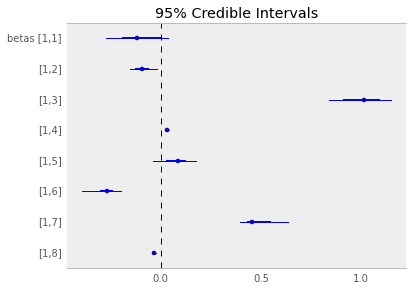In :
M_titanic.betas.summary()

betas:

Mean             SD               MC Error        95% HPD interval
------------------------------------------------------------------
-0.103           0.103            0.01             [-0.27   0.035]
-0.093           0.038            0.004            [-0.153 -0.019]
0.993            0.1              0.01             [ 0.837  1.145]
0.033            0.006            0.0              [ 0.023  0.044]
0.077            0.062            0.006            [-0.04   0.174]
-0.271           0.047            0.005            [-0.39  -0.198]
0.484            0.071            0.007            [ 0.395  0.634]
-0.031           0.003            0.0              [-0.037 -0.025]

Posterior quantiles:

2.5             25              50              75             97.5
|---------------|===============|===============|---------------|
-0.27            -0.189          -0.119         0.004         0.036
-0.173           -0.123          -0.093         -0.064        -0.025
0.828            0.912           1.013          1.086         1.141
0.022            0.03            0.034          0.037         0.044
-0.039           0.03            0.085          0.124         0.178
-0.38            -0.297          -0.268         -0.242        -0.175
0.396            0.434           0.458          0.545         0.637
-0.037           -0.033          -0.031         -0.029        -0.025